# Softmax + CE v/s Sigmoid + BCE for Single Label Classification

Hey everybody!

I have been following the fastbook reading group hosted by aman arora from wandb and in the exercises of #fastbook, in chapter 6 there’s a question which asks the reader to compare the performance of a single label classification (SLC) problem using CE loss and BCE Loss (i.e. single label and multi-label classification (MLC) approaches for a problem which only needs you to predict one label per datapoint).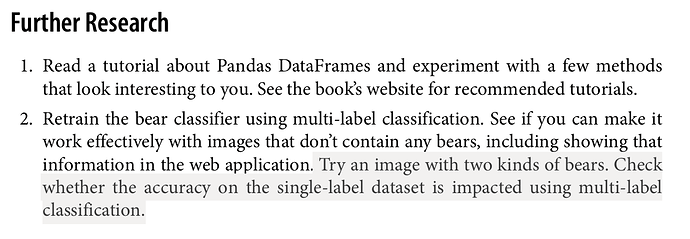# Model Building & Evaluation

I tried running an experiment on the `URLs.PETS` dataset of `fastai` and I have observed some interesting things which I wanted to share. I used same training and validation data for building two classification models.

1. Single Label Classifier with softmax activation and CE loss.
2. Multi Label Classifier with sigmoid activation and BCE loss. (With thresholding and selection of the best threshold i.e. where accuracy is maximum)

The experiment code is available here. I wanted to just show the results and ask a few questions to the community based on my observations and wanted to know your thoughts.

On the surface it seems MLC (right) outperforms SLC (left)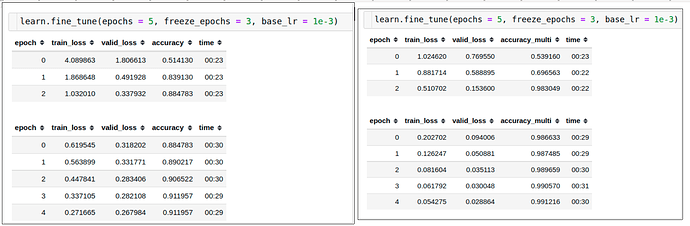However, on close inspection of the two I observed that the high accuracy of MLC can be attributed to the fact that it is also giving weightage at any given datapoint to all the classes which were not actually the label for that datapoint i.e.

``````groundtruth = [1, 0, 0, 0, 0]
slc_probas = [0.3, 0.1, 0.1, 0.5]
mlc_probas = [0.7, 0.8, 0.3, 0.2]

slc_prediction = argmax(slc_probas) = 3
mlc_prediction = [1, 1, 0, 0] # Assuming threshold = 0.5

slc_accuracy = 0
mlc_accuracy = 3 / 4 = 0.75
``````

This is why there seems to be a huge hike in the accuracy in MLC. If we were to make the criterion stricter i.e. in MLC, we compare the predictions for all classes in a datapoint and aggregate the matches later, we get a different picture.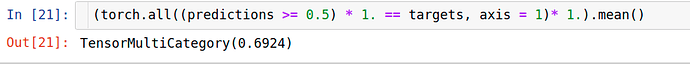Q1: Why does sigmoid activation + binary cross entropy perform so poorly as compared to softmax activation + cross entropy for single label classification? Both loss functions take all the 37 output activations in account; however one squeezes them to be in 0-1 range whereas the other doesn’t impose that restriction. Does that make such a great difference?

# Error Analysis

I have created two dfs for each approach which could be found here. The one for multi-label classification looks as follows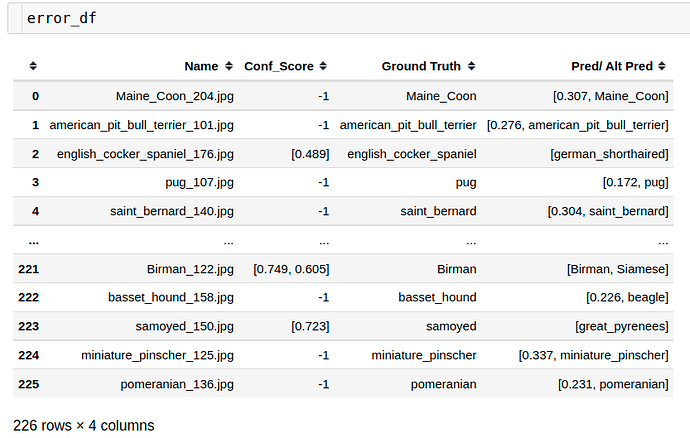PS: Here, `-1` in `Conf_score` column indicates no class was predicted for this name. For such cases, we tried to predict alternatively using `argmax` approach which is not advisable in a multi-label classification problem.

There’s 226 out of 920 which were problematic. What are the kind of problems encountered?

1. For the best threshold, some datapoints don’t get assigned a class at all i.e. such a prediction means the given datapoint doesn’t have any cat/dog of the mentioned breed. 0, 1 for eg. in above snippet
2. Multiple breeds are predicted for a single image for the best threhsold. 221 for eg.
3. Different/Wrong breed gets predicted for an image. 2 for eg.

On the other hand for single label classification, we get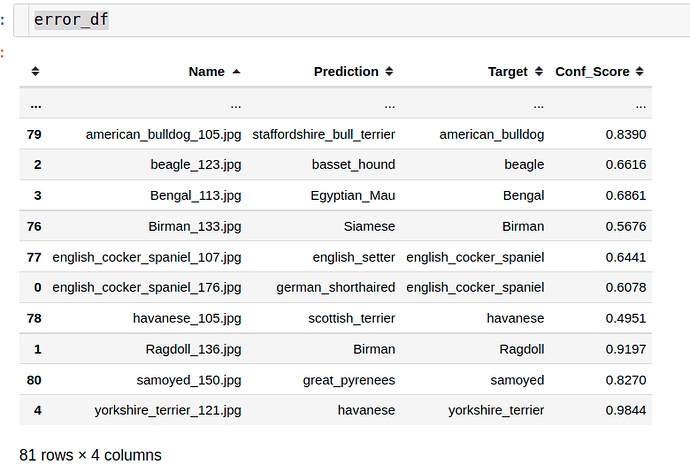Here we see there’s only 81 out of 920 which are mispredicted i.e. `target != prediction`.

But if we look at the confidence scores of the mispredictions, they’re pretty high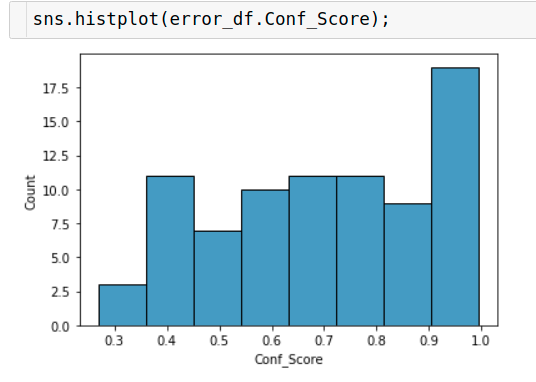We see that almost in half of the mispredictions that the model made, it was more than 70% confident that it is right. Now, this is dangerous because if we ideally want to use the mode it shouldn’t be overconfident on it’s mispredictions. This at least would give us an outlet to say if the confidence score of the prediction is less than 50% or 40% or whatever, don’t trust the model’s prediction or take it with a pinch of salt let’s say.

Q2: The model using softmax activation with cross entropy loss is overconfident of it’s mispredictions. How to tune it’s probability scores so that during inference, when doing argmax, if the probability is less than 50% for the most probable class, we can inform the end-user to use the inference label with a caveat.

Thanks,
Vinayak.